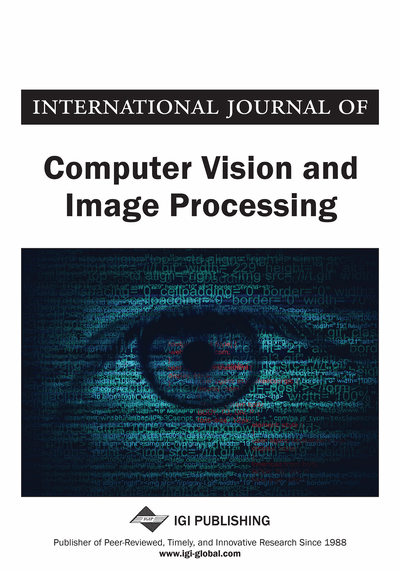# Three-Dimensional Face Shape by Local Fitting to a Single Reference Model

Rubén García-Zurdo (Colegio Universitario “Cardenal Cisneros”, Madrid, Spain)
Copyright: © 2014 |Pages: 13
DOI: 10.4018/ijcvip.2014010102

## Abstract

The authors present a simple method to estimate the three-dimensional shape of a face from an input image using a single reference model, based on least squares between the output of the linear-nonlinear (LN) neuronal model applied to blocks from an intensity image and blocks from a depth reference model. The authors present the results obtained by varying the LN model parameters and estimate their best values, which provide an acceptable reconstruction of each subject's depth. The authors show that increasing the light source angle over the horizontal plane in the input image produces slight increases in reconstruction error, but increasing the ambient light proportion produces greater increases in reconstruction error. The authors applied the method to predict each subject's unknown depth using different individual reference models and an average reference model, which provides the best results. As a noise reduction technique, the authors perform a point by point weighted averaging with the average reference model with weights equal to the fractions of the squares of the Laplacian of a Gaussian applied to the prediction and to the reference depth over the sum of both. Finally, the authors present acceptable visual results obtained from external images of faces under arbitrary illumination, having performed an illumination estimation previously.
Article Preview

## Introduction

Shape from shading is usually presented as an ill-posed problem, and although attempts to present the problem as well-posed exist (Prados & Faugeras, 2005), it is necessary to employ constraints of some kind in order to find a proper solution. A class of such constraints is obtained by applying dimensionality reduction techniques to large samples of images, usually faces, and their corresponding surfaces. In this context it is considered that there is a low ratio between the sample size and the number of variables needed to apply these techniques (Castelán, Puerto-Souza & Van Horebeek, 2009). Among such techniques are principal component analysis (PCA) applied to depth images (Atick, Redlich & Griffin, 1996), PCA applied to depth and texture images separately (Blanz & Vetter, 1999), PCA applied to coupled intensity and depth images (Castelán, Smith, & Hancock, 2007), searching for latent variables by partial least squares (Castelán & Van Horebeek, 2008) and canonical correlation (Reiter et al., 2006; Castelán et al., 2009).

From another point of view, the face molding method (Kemelmacher & Basri, 2006; Kemelmacher-Shlizerman & Basri, 2011) uses the surface and albedo of a single reference model to find a solution that simultaneously satisfies the reflectance equation for the input image and its similarity to the surface and albedo of the reference model. It does so by applying two regularization terms, one for the difference between the predicted surface and the reference surface, and another one for the difference between the predicted albedo and reference albedo, formulated as a Laplacian of a Gaussian to assure that the difference between the prediction and the model is smooth. To obtain the image surface, which acts as the unknown term in the model, it appeals to the Lambertian reflectance equation using the reference model albedo and a vector of estimated illumination of the image in the form of spherical harmonic coefficients. By using a first order approximation of four spherical harmonics (Kemelmacher & Basri, 2006) at least 87.5% of the variability of energy in the image is captured (Basri & Jacobs, 2003). With a second order approximation of nine spherical harmonics (Kemelmacher-Shlizerman & Basri, 2011) at least 99.2% of the variability of energy in the image is captured (Basri & Jacobs, 2003). Similar reconstruction results are obtained with both approaches, although the first order one of four coefficients is faster and accurate enough, with an average error in illumination estimation of 11.3 ° (Kemelmacher & Basri, 2006). The four coefficients of the first order approximation are estimated by least squares from the set of linear equations that relate the surface normals and albedo of the reference model to the test image. It is equivalent to a description of the illumination by a point source at infinity plus a homogeneous ambient lighting. A drawback of this method is the need for an appropriate reference model for the test image. Kemelmacher and Basri (2011) compared the average of the entire sample of subjects as a reference model to using each of the individual subjects as a reference model, obtaining better results for the average model compared to individual models in the form of mean error.

## Complete Article List

Search this Journal:
Reset
Open Access Articles: Forthcoming
Volume 10: 4 Issues (2020): Forthcoming, Available for Pre-Order
Volume 9: 4 Issues (2019): 2 Released, 2 Forthcoming
Volume 8: 4 Issues (2018)
Volume 7: 4 Issues (2017)
Volume 6: 2 Issues (2016)
Volume 5: 2 Issues (2015)
Volume 4: 2 Issues (2014)
Volume 3: 4 Issues (2013)
Volume 2: 4 Issues (2012)
Volume 1: 4 Issues (2011)
View Complete Journal Contents Listing